# Creating 3 Stacks With 1 Array in JavaScriptIn this article, you'll learn how to create 3 Stacks with 1 Array in JavaScript

## What Is A Stack?

A stack is a data structure that is based on the concept of “last-in-first-out” or “LIFO.” You can think of it like a stack of books where the top book has to be taken off the stack before you can retrieve the bottom book. JavaScript doesn’t have a native stack data structure, so we’re going to create one today.

Our array will contain three different stacks of a fixed size. The top of the stack will be on the right side and the bottom of the stack will be on the left side. You can picture it similar to this diagram. If this stack was full, the bottom element would live at `stack`and the top element would live at `stack[stack.length-1]`.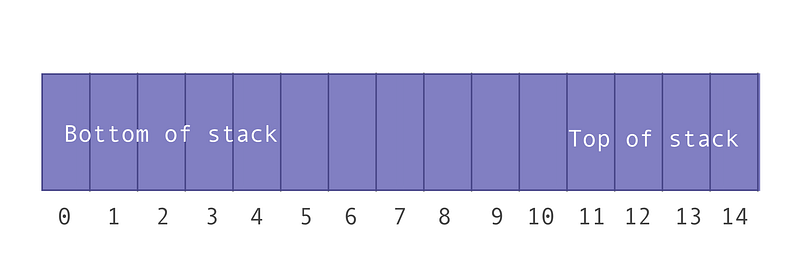### Class Breakdown

Our stacks will have a fixed size which will be equal to the argument passed in at instantiation.

#### Properties

The following properties will be initialized within the constructor:

• _stackCapacity: The maximum number of elements that can fit into one stack. This is a read-only property which is why it’s prepended with an underscore.
• values: An array which contains of all elements in the three stacks
• sizes: An array with three indices, each representing the current number of elements in the respective stacks.
• numberOfStacks: A constant which represents the total number of stacks we’ll allow our array to hold. We’re initializing this to three, however future iterations of this MultiStack class could take in a second argument to customize the number of stacks the array can hold.

#### Methods

Our MultiStack class will contain the following methods:

• get stackCapacity(): Returns the total capacity of each of the stacks (this is just a way for me to check that everything is working as expected, we won’t really be using this.)
• push(stackNumber, value): Pushes the value onto the top of the respective stack number.
• pop(stackNumber): Pops the top item off of the respective stack number.
• peek(stackNumber): Returns the top item off of the respective stack number. It’s just a way for us to “peek” at what element is on the top; no stack mutation will happen.
• isEmpty(stackNumber): Returns a boolean which indicates whether the respective stack has values.
• isFull(stackNumber): Returns a boolean which indicates whether the respective stack is full.
• indexOfTop(stackNumber): A helper method which returns the index, in the values array, of the top element in the respective stack.

#### Constructor

The first thing we’ll do is create our constructor. It will take in one argument, the stack size. Thus, the total length of our values array will be 3 * the stack size (since we’re initializing numberOfStacks to three).

We will initialize the sizes array to contain three indices with the value zero. For our purposes we will assume that the values being pushed onto the stacks are positive integers. You can change this logic to fit your needs.

``````class MultiStack {
constructor(stackSize) {
this._stackCapacity = stackSize;
this.values = [];
this.sizes = [0, 0, 0];
this.numberOfStacks = 3;
}
}
``````

#### Get Stack Capacity

This method returns the total capacity of each of the stacks (this is just a way for me to check that everything is working as expected, we won’t really be using this.)

``````get stackCapacity() {
return this._stackCapacity;
}
``````

#### isFull

This method returns a boolean which indicates whether the respective stack is full. It will check how many elements are currently on the respective stack and compare it against the stack capacity.

``````isFull(stackNumber) {
return this.sizes[stackNumber] === this._stackCapacity;
}
``````

#### isEmpty

This method returns a boolean which indicates whether the respective stack has values.

``````isEmpty(stackNumber) {
return this.sizes[stackNumber] === 0;
}
``````

#### indexOfTop

This is a helper method which returns the index, in the values array, of the top element in the respective stack.

This explanation could get a little tricky, so stick with it! I’ve included diagrams to better visualize the process.

First we need to grab the offset of the stack within the values array. To do this, we’ll multiply the stack number we want by the capacity of each stack.

For example, let’s find the index of the top item in stack 2 given that the `_stackCapacity` for each stack is 5. The stacks contain the following elements:

• Stack 0: [1, 12]
• Stack 1: [18, 8, 2]
• Stack 2: [5, 9, 66, 15]

Here is a visual representation of what the values array looks like: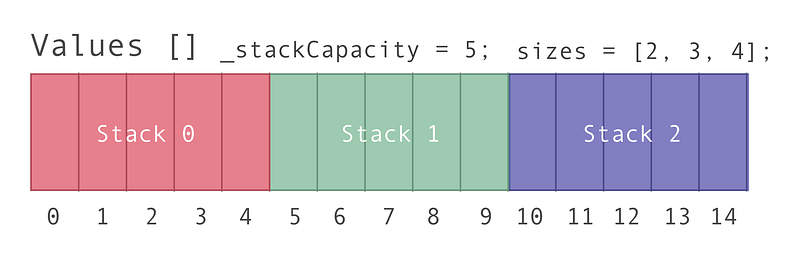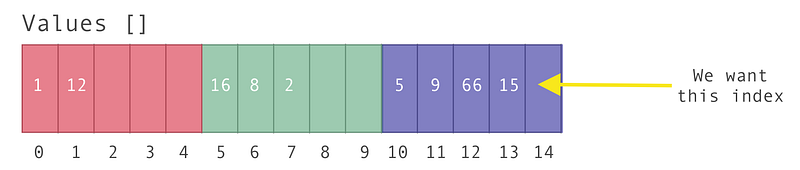Step 1: Calculate the offset; find the index of the bottom item in stack two

Assuming our stacks start at zero (i.e. stack 0, stack 1, stack 2), we can find where the bottom of stack two starts in the values array by multiplying the stack we’re looking for, two, by the stack capacity, which is the value passed in at instantiation. If our stack capacity is five, we know that the bottom element of stack two starts at index 10 in the values array.

index of bottom element in stack 2 = stack we’re looking for * capacity of each stack.

index of bottom element in stack 2 = 2 * 5 (found from `<em>_stackCapacity</em>`)

index of bottom element in stack 2 = 10.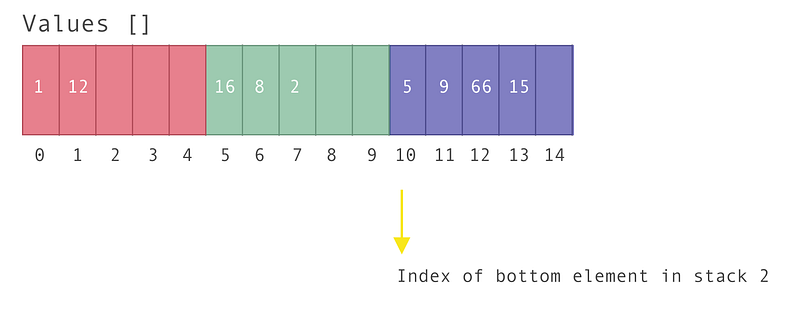Step 2: Calculate the total number of values currently in stack two

We already know how many values are in stack 2; they’re being kept in the `sizes` array. So by grabbing the value of `sizes` we know how many elements are in stack 2: 4

Step 3: Add the offset with the total number of values in the stack, minus one

We have to subtract one from the number of items in the stack, since our array starts at index zero.

When we add it all up we get:

index of top element in stack 2 = offset + number of values in stack two— 1

index of top element in stack 2 = 10 + 4 — 1

index of top element in stack 2 = 13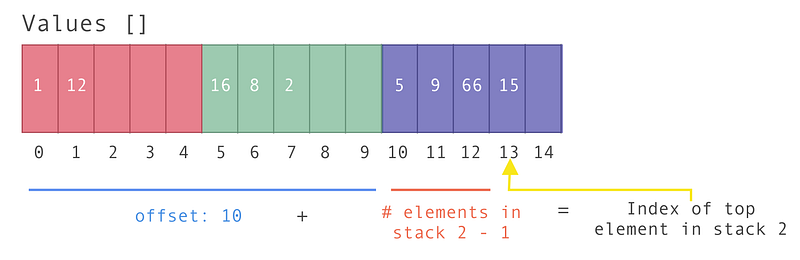The code for this is as follows:

``````indexOfTop(stackNumber) {
const offset = stackNumber * this._stackCapacity; // Find the starting point in the array
const size = this.sizes[stackNumber]; // How many elements are in that stack currently?
return offset + size - 1;
}
``````

#### Push

The push method pushes a value onto the top of the respective stack. It takes in two arguments:

• The stack to push the value onto
• The value
1. The first thing we have to do is check whether the stack is full. If it is full, let’s `console.log` the message `Stack number \${stackNumber} is full`.

2. If the stack isn’t full, increase the number of items in the stack, which is found in the `sizes` array.

3. Then add the new value to the top of the stack. We’ll use the `indexOfTop`method we just explained above to grab the top of the stack and add a value on top of it.

4. If it’s successfully added, let’s `console.log` a friendly message.

``````push(stackNumber, value) {
if (this.isFull(stackNumber)) {
return console.log(`Stack number \${stackNumber} is full`);
}
// Add one to the respective stack count
this.sizes[stackNumber]++;
// Add the value to the list
this.values[this.indexOfTop(stackNumber)] = value;
return console.log(`Item \${value} has been successfully added to stack \${stackNumber}`);
}
``````

#### Pop

This method pops the top item off of the respective stack number. It takes in one argument:

• The stack to pop the value off of
1. We must first check if the stack is empty using the `isEmpty` method. If it is, we’ll return a `console.log` a message.
2. If the stack isn’t empty, we’ll grab the index of the top element on the stack using the `indexOfTop` method and save it to a variable called `topIndex`.
3. Now let’s grab the value of that element. We can do this with `this.values[topIndex]` . We’ll return this element, which is why we need to save it to a variable.
4. We also need to tell the values array that the value at this index no longer exists. We’ll set this explicitly to zero (this could pose issues if your stack can take zero as a value, but for our sake we’ll assume the stack only accepts positive integers).
5. We must also decrement the size of the stack in the sizes array. We can do this with `this.sizes[stackNumber]--`.
6. Finally, let’s return the value we just popped off.
``````pop(stackNumber) {
if (this.isEmpty(stackNumber)) {
return console.log(`Stack number \${stackNumber} is empty`);
}
const topIndex = this.indexOfTop(stackNumber);
const value = this.values[topIndex];
this.values[topIndex] = 0; // Clear out element
this.sizes[stackNumber]--; // Reduce num elements in the stack
return value;
}
``````

#### Peek

This method returns the top item off of the respective stack number. It doesn’t alter the stack, it simply lets you view the element on the top. It takes in one argument:

• The stack whose top value we want to peek at
1. We first have to check if the stack is empty. We can use the `isEmpty`method to do so. If it is empty, let’s `console.log` a friendly message.
2. If the stack isn’t empty, we need to find the index for the element on top of the stack. We can use the `indexOfTop` method to do so.
3. Finally, we can return the value found at that index with `this.values[topIndex]` .
``````peek(stackNumber) {
if (this.isEmpty(stackNumber)) {
console.log(`Stack number \${stackNumber} is empty`);
}
const topIndex = this.indexOfTop(stackNumber);
return this.values[topIndex];
}
``````

## Putting It All Together

The final class looks like this:

``````class MultiStack {
constructor(stackSize) {
this._stackCapacity = stackSize;
this.values = []; // values in the stack which is max size of stackSize * 3
this.sizes = [0,0,0]; // length of 3 and each index contains the number of items in the respective stack
this.numberOfStacks = 3;
}

get stackCapacity() {
return this._stackCapacity;
}

push(stackNumber, value) {
if (this.isFull(stackNumber)) {
return console.log(`Stack number \${stackNumber} is full`);
}
// Add one to the respective stack count
this.sizes[stackNumber]++;
// Add the value to the list
this.values[this.indexOfTop(stackNumber)] = value;
return console.log(`Item \${value} has been successfully added to stack \${stackNumber}`);
}

pop(stackNumber) {
if (this.isEmpty(stackNumber)) {
return console.log(`Stack number \${stackNumber} is empty`);
}
const topIndex = this.indexOfTop(stackNumber);
const value = this.values[topIndex];
this.values[topIndex] = 0; // Clear out element
this.sizes[stackNumber]--; // Reduce num elements in the stack
return value;
}

peek(stackNumber) {
if (this.isEmpty(stackNumber)) {
console.log(`Stack number \${stackNumber} is empty`);
}
const topIndex = this.indexOfTop(stackNumber);
return this.values[topIndex];
}

isEmpty(stackNumber) {
return this.sizes[stackNumber] === 0;
}

isFull(stackNumber) {
return this.sizes[stackNumber] === this._stackCapacity;
}

indexOfTop(stackNumber) {
const offset = stackNumber * this._stackCapacity; // Find the starting point in the array
const size = this.sizes[stackNumber]; // How many elements are in that stack currently?
return offset + size - 1;
}
}
``````

You’ve now created an array that represents three fixed-size stacks! You can view the CodePen for this class here.

## The essential JavaScript concepts that you should understand

The essential JavaScript concepts that you should understand - For successful developing and to pass a work interview

## Grokking Call(), Apply() and Bind() Methods in JavaScript

In this article, we will have a look at the call(), apply() and bind() methods of JavaScript. Basically these 3 methods are used to control the invocation of the function.

## JavaScript Tutorial: if-else Statement in JavaScript

This JavaScript tutorial is a step by step guide on JavaScript If Else Statements. Learn how to use If Else in javascript and also JavaScript If Else Statements. if-else Statement in JavaScript. JavaScript's conditional statements: if; if-else; nested-if; if-else-if. These statements allow you to control the flow of your program's execution based upon conditions known only during run time.

## How to Retrieve full Profile of LinkedIn User using Javascript

I am trying to retrieve the full profile (especially job history and educational qualifications) of a linkedin user via the Javascript (Fetch LinkedIn Data Using JavaScript)

## JavaScript Tutorial 50 - Promises in JavaScript

JavaScript Tutorial 50 - Promises in JavaScript - This tutorial describes how to create and handle promises in JavaScript.